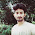### Previous GATE Questions on K-Map, SOP and POS expressions (1987 - Till Date)

1991

1.       The four variable function f is given in terms of min-terms as f(A, B, C, D) = m(2,3,8,10,11,12,14,15). Using the K-map minimize the function in the sum of products form. Also, give the realization using only two input NAND gates.

Answer:     f = AD' + AC + B'C and No.of NAND gates required = 6

1993

1.       The truth table for the output Y in terms of three inputs A, B and C are given. Draw a logic circuit realization using only NOR gates.
Answer:      Y = (A' + B')(B' + C')(A' + C')

1996

1.       Given the Boolean function F in three variables R, S and T as

F = R’ST’ + RS’T + RST
a.       Express F in the minimum sum of products form
b.      Express F in the minimum product of sums form
c.       Assuming that both true and complement forms of the input variables are available,     draw a circuit to implement F using the minimum number of 2 input NAND gates only.

1997

1.   The Boolean function A + BC is a reduced form of
a.       AB + BC
b.      (A + B)(A + C)
c.       A’B + AB’C
d.      (A + C)B

1998

1.   The K – map for a Boolean function is shown in the figure. The number of essential prime implicants for this function is
a.       4
b.      5
c.       6
d.      7

2.       For the identity, AB + A’C + BC = AB + A’C, the dual form is

1999

1.       The logical expression Y = A + A’B is equivalent to
a.       AB
b.      A’B
c.       A’ + B
d.      A + B

2.       The minimized form of the logical expression

2003

1.       The number of distinct Boolean expressions of 4 variables is
a.       16
b.      256
c.       1024
d.      65536

2.       If the functions W, X, Y and Z are as given below,

2004

1.       The Boolean expression AC + BC’ is equivalent to

2005

1.       The Boolean expression for the truth table shown is

2006

1.       The number of product terms in the minimized sum of product expression obtained through the following K-map (where “d” denotes don’t care states)
 1 0 0 1 0 d 0 0 0 0 d 1 1 0 0 1
a.       2
b.      3
c.       4
d.      5

2007

1.       The Boolean function Y = AB + CD is to be realized using only 2 input NAND gates. The minimum number of gates required is
a.       2
b.      3
c.       4
d.      5

2.       The following Boolean expression Y = A’B’C’D +  A’BCD’ + AB’C’D + ABC’D’

can be minimized to

2009

1.       If X = 1 in the given logic equation, then
a.       Y = Z
b.      Y = Z’
c.       Z = 1
d.      Z = 0

2012

1.       In the sum of products function, f(X,Y,Z) = (2,3,4,5), the prime implicants are

2014

1.       The Boolean expression simplifies to

2.       For the given Boolean function, which one of the following is the complete set of essential prime implicants?

1.2.1.3.4.Superb one it is

5.college ka exam dete dete dekh lena yehi se aayega

6.